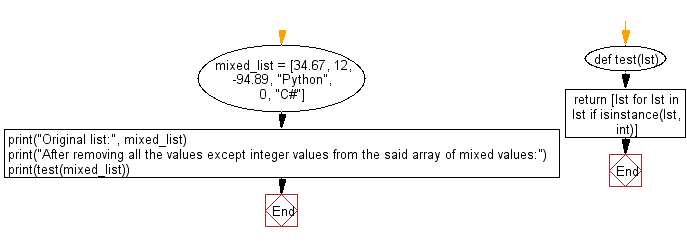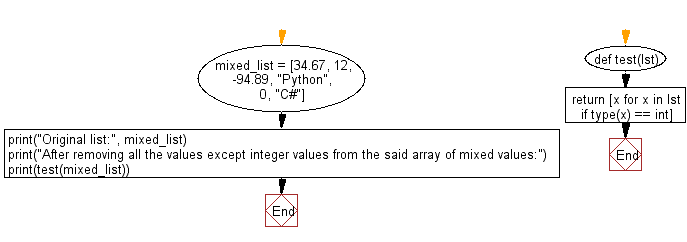﻿ Python: Remove all the values except integer values from a given array of mixed values - w3resource# Python: Remove all the values except integer values from a given array of mixed values

## Python List: Exercise - 212 with Solution

Write a Python program to remove all the values except integer values from a given array of mixed values.

Sample Solution-1:

Python Code:

``````def test(lst):
return [lst for lst in lst if isinstance(lst, int)]
mixed_list = [34.67, 12, -94.89, "Python", 0, "C#"]
print("Original list:", mixed_list)
print("After removing all the values except integer values from the said array of mixed values:")
print(test(mixed_list))
```
```

Sample Output:

```Original list: [34.67, 12, -94.89, 'Python', 0, 'C#']
After removing all the values except integer values from the said array of mixed values:
[12, 0]
```

Flowchart:## Visualize Python code execution:

The following tool visualize what the computer is doing step-by-step as it executes the said program:

Sample Solution-2:

Python Code:

``````def test(lst):
return [x for x in lst if type(x) == int]
mixed_list = [34.67, 12, -94.89, "Python", 0, "C#"]
print("Original list:", mixed_list)
print("After removing all the values except integer values from the said array of mixed values:")
print(test(mixed_list))
```
```

Sample Output:

```Original list: [34.67, 12, -94.89, 'Python', 0, 'C#']
After removing all the values except integer values from the said array of mixed values:
[12, 0]
```

Flowchart:## Visualize Python code execution:

The following tool visualize what the computer is doing step-by-step as it executes the said program:

Python Code Editor:

Have another way to solve this solution? Contribute your code (and comments) through Disqus.

What is the difficulty level of this exercise?

Test your Python skills with w3resource's quiz

﻿

## Python: Tips of the Day

```print(2_000_000)
```2000000